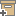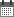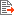Syntax
Search...
Manage
Community:
אסיף מאגר המחקר החקלאי
On the estimation of N mineralization parameters in incubation experimentsBack to searchPrevious item
Next item
Year:
1981
Authors :
Bar-Yosef, Bnayahu
;
.
Fine, Pinchas
;
.
Talpaz, Hovav
;
.
Volume :
45
Co-Authors:

H. Talpaz

P. Fine

B. Bar‐Yosef

Facilitators :
From page:
993
To page:
996
(
Total pages:
4
)
Abstract:

Accurate prediction of nitrogen mineralization in soils requires reliable parameters which are determined in incubation experiments. The estimated values of the potentially mineralizable organic nitrogen (No) and the mineralization rate constant (k) also depend on the mathematical analysis of the incubation results. Analysis of data by other researchers (Stanford and Smith, 1972) by a nonlinear regression procedure gave estimates of No and k which differed from those obtained by a linear regression technique. Theoretical considerations show that the difference between the two techniques results mainly from the specified behavior of the error term in the mineralization rate equation. The nonlinear regression procedure is shown to be preferable to the linear regression technique.

Note:
Related Files :
soil
Related Content
More details
DOI :
10.2136/sssaj1981.03615995004500050036x
Article number:
0
Affiliations:
Database:
Publication Type:
article
;
.
Language:
English
Editors' remarks:
ID:
52816
Last updated date:
02/03/2022 17:27
Creation date:
29/12/2020 23:34Scientific Publication
On the estimation of N mineralization parameters in incubation experiments
45

H. Talpaz

P. Fine

B. Bar‐Yosef

On the estimation of N mineralization parameters in incubation experiments

Accurate prediction of nitrogen mineralization in soils requires reliable parameters which are determined in incubation experiments. The estimated values of the potentially mineralizable organic nitrogen (No) and the mineralization rate constant (k) also depend on the mathematical analysis of the incubation results. Analysis of data by other researchers (Stanford and Smith, 1972) by a nonlinear regression procedure gave estimates of No and k which differed from those obtained by a linear regression technique. Theoretical considerations show that the difference between the two techniques results mainly from the specified behavior of the error term in the mineralization rate equation. The nonlinear regression procedure is shown to be preferable to the linear regression technique.

Scientific Publication
You may also be interested in